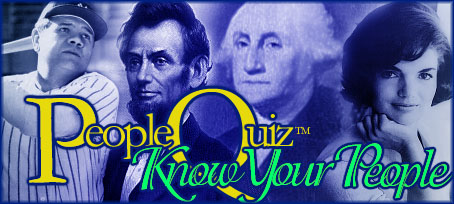.gsc-search-box { } Register for extra features!    LogonTrivia GamesBlogScoreboardsActivityCelebrity TriviaBiographiesPlayers
 Trivia Quiz - Mathematical Symbols How well do you know mathematical symbols? Quiz Number: 2006 Date Submitted: January 10, 2008 Quiz Categories: Science & Nature Quiz Type: General Quiz Author: BubblyJolie Average Score: 83.8 percent Times Taken: 162 times Taken by Registered Users: 20 Click here for the code snippet to embed this quiz in your website.(Image Source: Math Symbols)

Be sure to register and/or logon before taking quizzes to have your scores saved.

1. What does this mean? âˆ‘
A.   Infinity
B.   equal to
C.   sum of
D.   parallel

2. What does this mean? â‰
A.   equal to
B.   not equal to
C.   defined as
D.   approaches

3. What does this mean? â‰¡
A.   plus or minus
B.   defined as
C.   approaches
D.   parallel

4. What does this mean? â‰ˆ
A.   equal to
B.   not equal to
C.   parallel
D.   aproximately equal to

5. What does this mean? âˆž
A.   sum of
B.   absolute value
C.   less than
D.   infinity

6. What does this mean? â•‘
A.   perpendicular
B.   parallel
C.   less than
D.   infinity

7. What does this mean? â‰¥
A.   greater than or equal to
B.   infinity
C.   sum of
D.   equal to

8. What does this mean? â‰¤
A.   less than or equal to
B.   less than
C.   much less than
D.   approximately equal to

9. What does this mean? â†’
A.   approaches
B.   less than
C.   sum of
D.   plus or minus

10. What does this mean? Ã·
A.   equal to
B.   not equal to
C.   defined as
D.   divide by

peoplequiz.com®

Pine River Consulting 2022